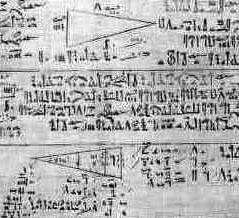# The frustrumIn the homework to the 21'st lecture, you have verified the formula ``` V = h (a2 + ab + b2)/3 ``` of the volume of the frustrum, the truncated pyramid. This example is historically very interesting because this formula appeared as Problem 14 in the Moscow Mathematical Papyrs whicih is believed to be written in 1850 BC. Remember that the trapezoid with base a and roof b and height h has area ``` A = h (a+b)/2 ``` So, it had been tempting to believe that the frustrum has volume ``` V = h (a2 + b2)/2 ``` especially because this gives the right answer h a2 if a=b.